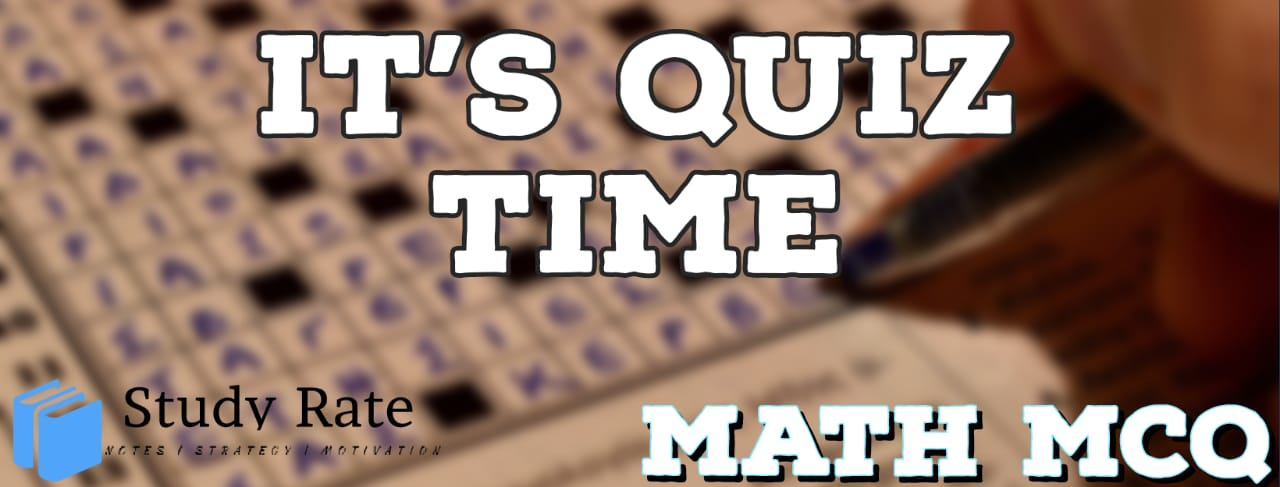## CBSE Class 10 Maths Chapter 13 – Surface Areas and Volumes MCQ Quiz

Free PDF Download of CBSE Class 10 Maths Chapter 13 – Surface Areas and Volumes Multiple Choice Questions with Answers. MCQ Questions for Class 10 Maths with Answers was Prepared Based on Latest Exam Pattern. Students can solve Class 10 Maths Surface Areas and Volumes MCQs with Answers to know their preparation level

CBSE MCQs Class 10 Maths Chapter 13 Surface Areas and Volumes

1. A cylindrical pencil sharpened at one edge is the combination of

2. The lateral surface area of a right circular cone of height 28 cm and base radius 21 cm(in sq. cm) is:

3. During conversion of a solid from one shape to another, the volume of the new shape will

4. A right circular cylinder of radius r cm and height h cm (h>2r) just encloses a sphere of diameter

5. A hollow cube of internal edge 22cm is filled with spherical marbles of diameter 0.5 cm and it is assumed that 1/8th space of the cube remains unfilled. Then the number of marbles that the cube can accommodate is

6. A solid metallic sphere of diameter 21 cm is melted and recast into a number of smaller cones, of diameter 3.5 cm and height 3cm. The number of cones so formed is:

7. An oil funnel made of tin sheet consists of a 10 cm long cylindrical portion attached to a frustum of a cone. If the total height is 22 cm, the diameter of the cylindrical portion is 8 cm and diameter of the top of the funnel is 18 cm, find the area of the tin sheet required to make the funnel.8. The radii of the top and bottom of a bucket of slant height 45cm are 28cm and 7 cm respectively. The curved surface area of the bucket is:

9. Twelve solid spheres of the same size are made by melting a solid metallic cylinder of base diameter 2 cm and height 16 cm. The diameter of each sphere is

10. The diameters of the two circular ends of the bucket are 44 cm and 24 cm. The height of the bucket is 35 cm. The capacity of the bucket is